# Lecture 2: Angles

Readings: Textbook pages 8th ed. 8 - 15 3rd Ed. 8 - 15

## Angular Diameter

• When we look at objects in the sky, we can't directly see how large they really are.
• All that we can measure is an apparent angle.
• When you look at the Moon, imagine a skinny triangle with the Moon's diameter as its base and the triangle's tip at your eyes.
 The angle at the triangle's tip (near your eyes) is 1/2 a degree. The Moon subtends an angle of 1/2 o. Define the angular diameter of the moon to be the angle that it subtends. Remember: short form for a degree = o. There are 360 o in a full circle.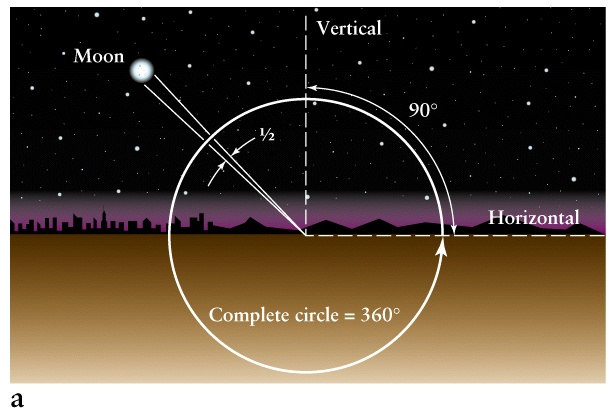Next

## Angular Separation of Stars

 When you look at the pointer stars of the Big Dipper, imagine a triange joining the pointer stars and your eyes. The angle at your eyes is 5o. In other words, the angular separation of the pointer stars is 5o. We do not know whether two stars are at the same distance from us or not (in contrast to two sides of the Moon), nor the relative distance between them. The angular separation is the only thing we determine from direct observations.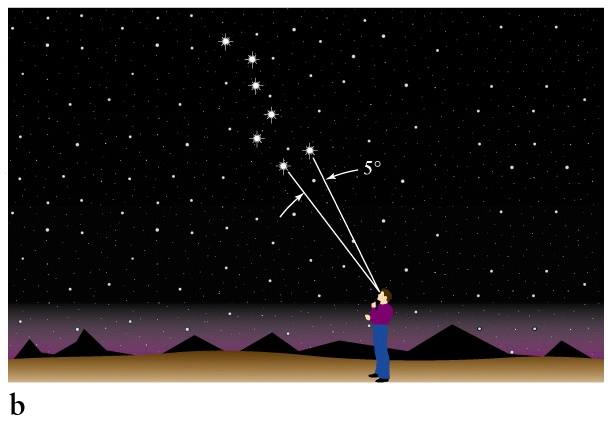Back Next

## Using Your Hands as a Protractor

 You can use your hands, held out at arms length, to estimate angles in the sky. Your smallest finger subtends an angle close to 1 o. If you spread your fingers and thumb out, the distance between your smallest finger and your thumb subtends an angle close to 20 o.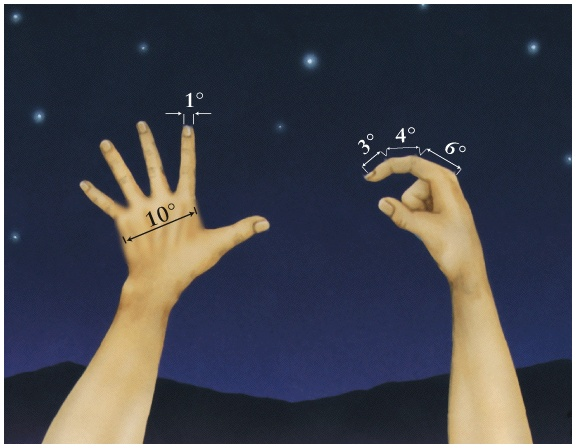Back Next

## Angular Diameter of Objects of Different Sizes

 Two objects which are different sizes might appear to have the same angular diameter because the smaller object is closer to us. For example, the Moon is much smaller than the Sun, but the Moon is also much closer than the Sun. This results in the coincidence that both the Moon and the Sun subtend the same angle (1/2 o) in the sky. This allows solar eclipses to occur!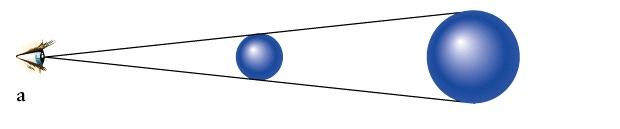Back Next

## A Solar Eclipse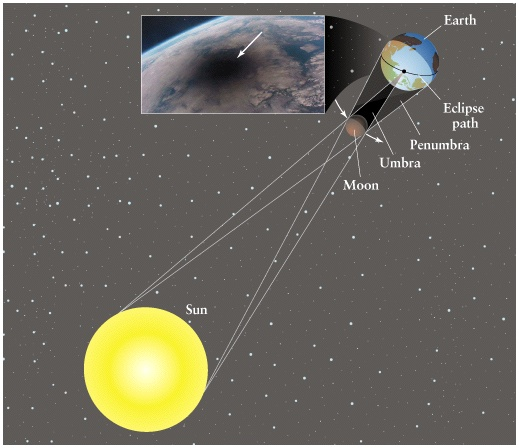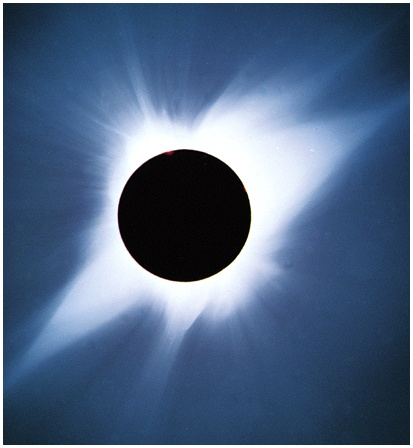Back Next

## Angular Diameter of Objects at Different Distances from the Observer

 Suppose that two identical objects are in the sky, but one is closer to us than the other. The object that is closer to us will subtend a larger angle than the further away object. One object will appear to be larger, although they are really the same size! If we know the size of the object, measuring angle will give us info about the distance !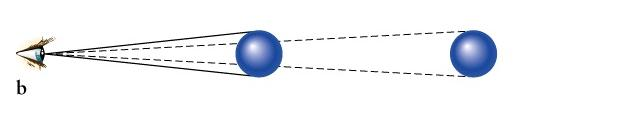Back Next

### Subdivisions of Degrees

• Often we need smaller angles in astronomy so a degree is subdivided into smaller angles.
• One degree is subdivided into 60 arc-minutes.
• The abbreviation for arc-minutes is '
• One arc-minute is subdivided into 60 arc-seconds.
• The abbreviation for arc-seconds is ''
• We have the following conversions:
1 o = 60 '
1 ' = 60 ''
• The conversion between degrees and arc-seconds is:
1o = 60 ' = 60 x 60 '' = 3600 ''

Back Next

• A fundamental angular unit used in mathematics is the radian.
• A radian is defined so that there are 2 &pi radians in a circle.
• Well, that sounds pretty arbitrary, isn't it ? More fundamentally, one radian is defined as the angle subtended by a circular arc of length equal the radius of the circle.
• Radians are important, because the small angle approximation is only true if the angle is given in radians.
• The small angle approximation is:

sin(A) = A ,     cos(A) = 1 ,     tan(A)=sin(A)/cos(A)=A

• Convert radians to degrees, arcminutes, and arcsec :
• 1 rad = 360o/ (2 &pi) = 57.2958o
• 1 rad = 3437.75 '
• 1 rad = 206265 ''

Back Next

## Small angle distance-size relation

 An object has a true diameter = D The distance to the object = d The angular diameter of the object in radians = Arad The angular diameter of the object in arc-sec = a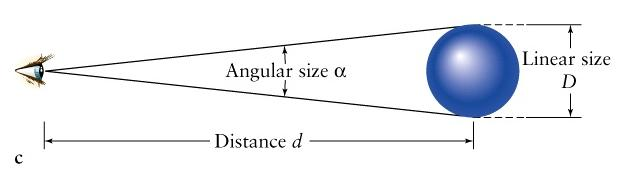Small angle formula:     D = d Arad
• But we usually measure angles in arc-sec.

Small angle formula in arcsec :     D = d a/206265

• Example: a ball has a diameter of 0.9 meters. From what distance it will have the same angular size as the star Sirius (0.007 '') ? Give the answer in meters using power of 10 notation to 3 significant digits (as is default in WebAssign !)
Solution: d = D*206265/a = 0.9*206265/0.007 = 2.65*107 m = 2.65e7 m

Back Next

## New Unit for Astronomical Distances - Parsec

 Viewed from a distance of 1 parsec the radius of Earth orbit , which is by definition 1AU, subtends the angle of 1 '', 1 parsec = 1 pc = 3.26 ly But why ??? Really parsec appears a natural unit when one considers effect of parallax as an observer on the Earth moves around the Sun When is it convenient to measure distances in parsecs ? Parallaxes of nearby stars are typically fraction of arcseconds, that's why a parsec is a natural unit to measure distances to them. The nearest star (Alpha Centauri) has a parallax of 0.746 '' and the distance 1.34 pc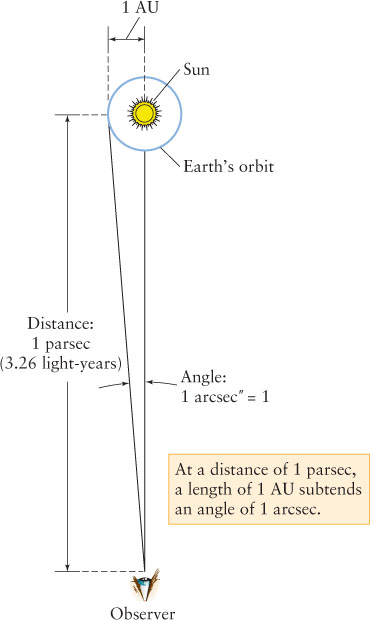Back Next

## Parallax: Change of the angular position of nearby object as observer moves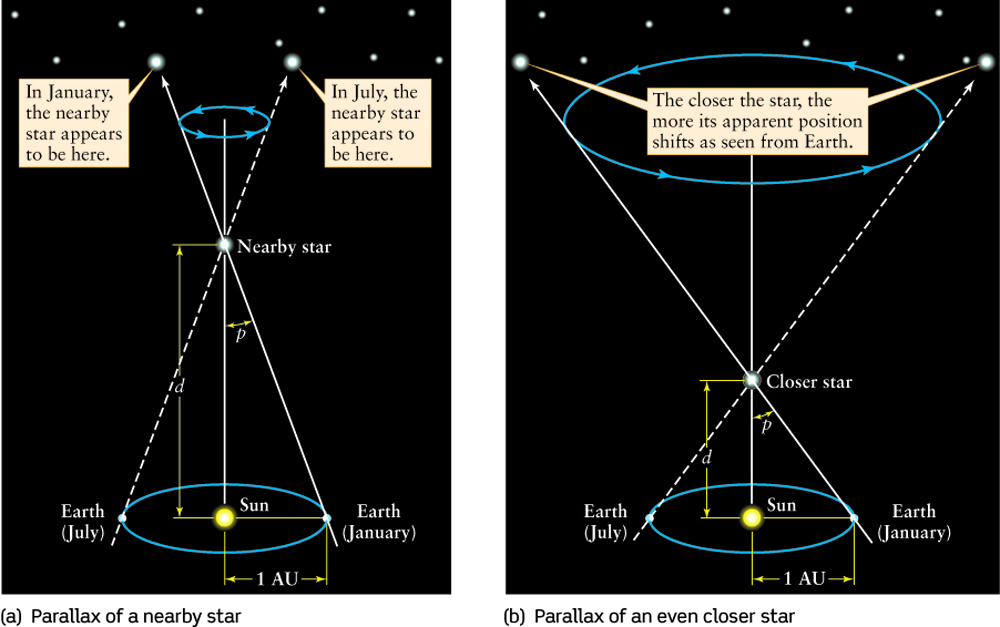Back

Next Lecture: Nature of Light
Read Chapter 5: 8th Ed. pages 97 - 124 or 3rd Ed. pages 97 - 124